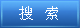说明：双击或选中下面任意单词，将显示该词的音标、读音、翻译等；选中中文或多个词，将显示翻译。 您的位置：首页 -> 句库 -> 算术及逻辑运算部件 1. arithmetic and logic unit data flow算术及逻辑运算部件数据流 2. register arithmetic logic unit寄存器运算逻辑部件 3. A Design of YHFT s Multiplier Unit and ALU;“银河飞腾”DSP乘法部件及算术逻辑运算部件的设计 4. The arithmetic logic unit (ALU), which performs arithmetic and logical operations.算术逻辑单元，用来进行算术逻辑运算。 5. The Design and Verification of a 600MHz YHFT-DX ALU600MHz YHFT-DX算术逻辑部件设计与验证 6. Data processing--Vocabulary--Section 02: Arithmetic and logic operationsGB/T5271.2-1988数据处理词汇02部分算术和逻辑运算 7. arithmetic logic register stack (ALRS)算术及逻辑寄存器栈 8. The central processing unit, or CPU, is the brain of a computer. It is where arithmetic and logical functions are performed. 中央处理器，或者说CPU，是计算机的大脑。是执行算术和逻辑（运算）功能的部件。 9. To perform the operation of negation.实现逻辑“非”运算。 10. 3.1.6 Logical operators3.1.6 逻辑运算符 11. Research and Full Custom Design of High Performance Arithmetic and Logical Unit;高性能算术逻辑部件研究与全定制设计 12. On the Mathematical Logic Operation "→" and BCK Operation "*;关于逻辑运算“→”和BCK运算“*” 13. The logical operation which makes use of the AND operator or logical product.使用AND运算符或逻辑乘积的逻辑运算。 14. calculator with arithmetic logic带有算术逻辑的计算器 15. arithmetic logic of pocket calculator袖珍计算机的算术逻辑 16. Arithmetic unit: That part of the computer which carries out calculations and makes logical decisions. 运算部:电脑进行计算和逻辑决定的部份。 17. The part in an instruction that specifies the kind of arithmetical or logical operation to be performed, but not the address of the operands. 指令中指明将要完成的算术运算或逻辑运算类型的那一部分,而不是操作数地址的部分。 18. A term sometimes used synonymously with" arithmetic(logic) unit" .本术语有时用作“运算（逻辑）器”的同义语。 ©2011 dictall.com